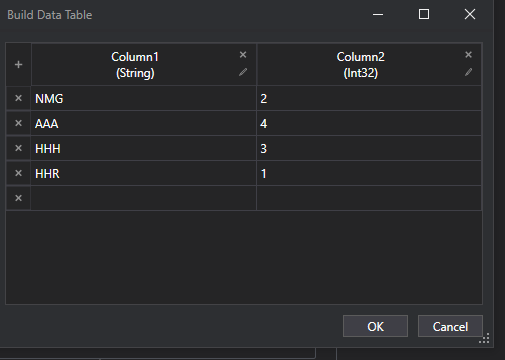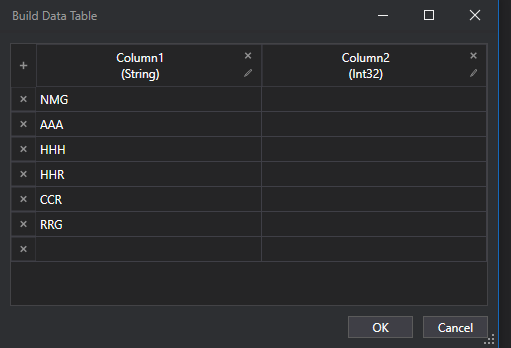# Compare Two Datatable using Linq

Hello Everyone,This is sample data table 1This is a satic data table where the rows are fixed.
Need to compare dt1 with static dt and merge column 2 and for the rest of table add value 0 zero if value is not available in dt1

Need a linq query for this scenario if possible.

Thank you

Try this,

use assign activity
left-> dt3
Right->(From dt1 In dt1.AsEnumerable
Join dt2 In dt2.AsEnumerable
On dt1(“Column1”).ToString Equals dt2(“Column1”).ToString
Select dt1).CopyToDataTable

but i need remaining rows too

dt_Static
[Column1,Column2
NMG,2
AAA,4
HHH,3
HHR,1
]
this is gives same output as dt1

d1.AsEnumerable.ToList.ForEach(Sub(r) r(1)=if(d.AsEnumerable.Where(Function(r1) r1(0).ToString.Equals(r(0).Tostring)).Count>0,CInt(d.AsEnumerable.Where(Function(r1) r1(0).ToString.Equals(r(0).Tostring)).First().Item(1)),0))

Hi @RVK ,

Have you checked the post below :

yes i checked this but for the remaning rows with no data needs to be appended with value 0

@RVK ,

Currently using the above expression results in the right number of rows but the Column2 is empty, is that right ?
If so, Try changing the Expression to the below :

``````(From d1 In dtData1.AsEnumerable
Group Join d2 In dtData2.AsEnumerable On d1(0) Equals d2(0) Into gj = Group
From g In gj.DefaultIfEmpty
Select ra = {d1(0) ,If(isNothing(g), CObj("0"), g(1)) }
``````

the case also can be handled with the Join DataTable activity

A LINQ approach could look like this:

Assign Activity
dtResult = dt2.Clone

Assign activity
dtResult =

``````(From dLeft In dt2.AsEnumerable
Group Join dRight In dt1.AsEnumerable
On dLeft(0).ToString.Trim Equals dRight(0).ToString.Trim Into gj = Group
From g In gj.DefaultIfEmpty
Let b = If(isNothing(g), 0, g(1))
Select ra = New Object() {dLeft(0), b }
``````

kindly note: we set the dt2 - dt Statis to the left side for a left join

then you can run this query to get the other values and fill the Column2 with zeros and append that data table with the other one

(Not(From dt1 In dt1.AsEnumerable
Join dt2 In dt2.AsEnumerable
On dt1(“Column1”).ToString Equals dt2(“Column1”).ToString
Select dt1)).CopyToDataTable

Thank you For the solution

This topic was automatically closed 3 days after the last reply. New replies are no longer allowed.